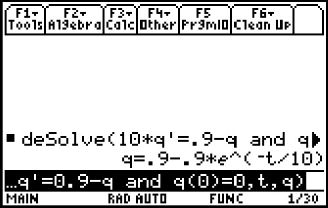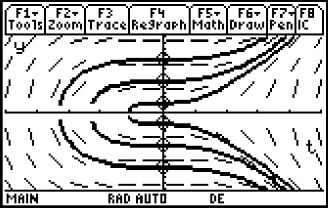# Activities

••• ##### Subject Area

• Math: Calculus: Slope Fields Analysis
• Math: Calculus: Differential Equations

• ##### Author9-12

20 Minutes

• ##### Device
• TI-89 / TI-89 Titanium

TI Connect™

• ##### Accessories

TI Connectivity Cable

• ##### Report an Issue

Charged Up#### Activity Overview

Students use the deSolve command to find general and particular solutions to differential equations and then dynamically explore the family of particular solution to a differential equation.

#### Key Steps

•Differential Equations are applicable for all sorts of physical phenomena, including radioactive decay, economics, biology, chemistry, population growth and electric circuits with capacitors. Students are asked some exam-like questions to introduce separable differential equations.

Students use deSolve to find general and particular solutions to differential equations.

•They will also graphically view the family of particular solutions to a differential equation.